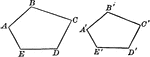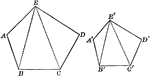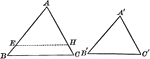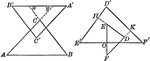### Similar Polygons (Pentagons) That Can Be Used To Show Proportionality

Illustration that shows similar polygons (pentagons) that can be used to show proportionality.### Similar Polygons (Pentagons) That Can Be Used To Show Proportionality

Illustration that shows similar polygons (pentagons) that can be used to show proportionality.### Mutually Equiangular Similar Triangles

Illustration that shows two mutually equiangular triangles are similar.### Similar Triangles With Respective Sides Parallel or Perpendicular

Illustration that shows that two triangles which have their sides respectively parallel, or respectively…### Similar Triangles With Altitudes Drawn

Illustration that shows two similar triangles with altitudes drawn.